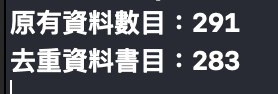#2019 iT 邦幫忙鐵人賽DAY 5
0

# 前言：# ＃計算折扣 & Debug

（ 內有踩雷過程，小心服用 ）

``````var discount: Int? {
if originPrice != 0 && sellPrice {
// 我們希望這兩者都不為 0，若是其中一個不存在就返回 nil。
let result = Int((Double(sellPrice) / Double(originPrice)) * 100.0)
return result
} else {
return nil
}
}
``````

``````for book in books {
print("原價:",book.originPrice, "售價:",book.sellPrice)
// 若是 book.dicount == nil，則會印出無折扣。
print("折扣:", book.discount ?? "無折扣")
}
``````這時候我們發生了突發狀況！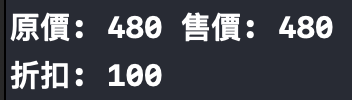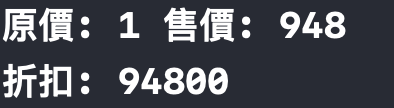What the hell ???通常我們在接來自後端的資訊或是 API 的時候，我們往往不知道他回傳的東西是不是我們想要的，所以如果後端能夠與我們合作那當然是很棒的，我們可以溝通我們需要怎麼樣的資訊，但如果今天是個 OpenData 是一個開放式的資料 API，那…

• 售價原價都有，但售價原價不同。
• 沒有原價，只有售價。（ 也就是沒有打折 ）

``````var discount: Int? {
// 我們希望這兩者都不為 0 時才能夠計算折扣，若是其中一個不存在就返回 nil。
if originPrice != 0 && sellPrice != 0 && originPrice > sellPrice {
let result = Int((Double(sellPrice) / Double(originPrice)) * 100.0)
return result
} else {
return nil
}
}
``````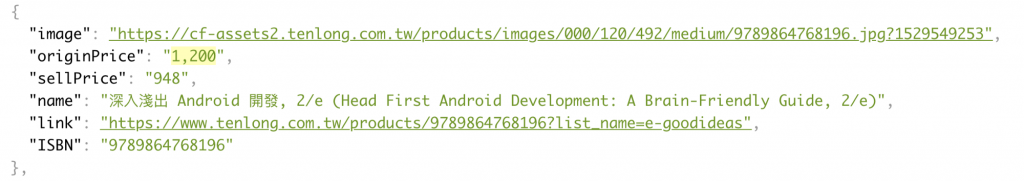``````// 舉個範例給大家看
// 我先 extension 我們的String，並新增一個回傳 Double 的方法。
extension String {
func toDouble() -> Double {
let double = (self as NSString).doubleValue
return double
}
}
let string1 = "12.34"
let string2 = "1,234.56"
let string3 = "1.234B56"
print(string1.toDouble()) // 12.34
print(string2.toDouble()) // 1.0
print(string3.toDouble()) // 1.234
``````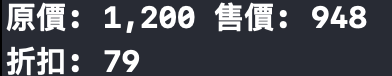??? 你以為這樣就結束了嗎？？ ???

``````let deduplicationBooks = Array(Set(books))
print("原有資料數目：\(books.count)")
print("去重資料書目：\(deduplicationBooks.count)")
``````